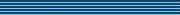### Schools Mathematics Grand Challenge

Tip of the Week

A geometric sequence is a list of numbers where the ratio of adjacent numbers in the list is always the same. For example, this is a geometric sequence:

6, 12, 24, 48, 96, ...

where the ratio is two. The ratio does not need to be a whole number - if we start at 6 and use a ratio of 2/3, then we get the sequence:

6, 4, 8/3, 16/9, ...

Geometric sequences often come up in compound interest, probability, and when estimating what more complicated sequences do.